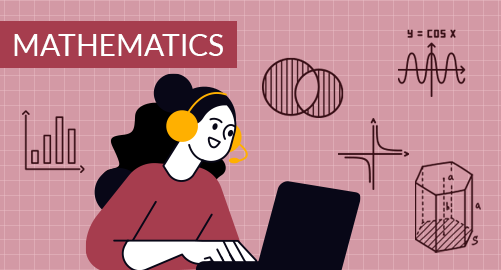#### through a demo today# Year 6 Mathematics

Mathematics
Year 6

The Year 6 Mathematics curriculum equips the students with various skills that are essential for mathematical development, understanding, and applying mathematical concepts for real-life situations and problem-solving. It covers topics from numbers, fractions, decimals, ratios and percentages, measurements, geometry, data handling, probability, and algebra.

## What you'll learn

• 1

Number System

• 2

Factors and Multiples

• 3

Fractions and Decimals

• 4

Ratio and Percentage

• 5

Geometry

• 6

Measurements

• 7

Data Handling and Probability

• 8

Time and Temperature

• 9

Algebra

## Skills you'll learnNumeracy Skills - Working with numbers, place values, and number operations.Problem-Solving Skills - Breaking down complex problems, solving real-life problems, and making logical decisions.Spatial and Geometrical Skills - Properties of 2D & 3D shapes, angles, symmetry, transformations, and creating maps, grids, and coordinates.Data Analysis and Interpretation - Data collection and organising using tables, graphs, and charts. Calculate and measure central tendency (mean, median, and mode).Measurement skills - Measuring length, area, volume, capacity, mass, and temperature. Conversion units and measurements.Algebraic Thinking - Understanding algebraic expressions and equations. Solving equations and inequations.Arithmetic Operations - Basic arithmetic operations on fractions, decimals, ratios, and percentages. Relationship between fractions, decimals, and percentages.Critical Thinking and Reasoning - Develop logical thinking, making logical connections, and evaluating strategies.Financial Literacy - Understanding basic financial concepts, calculating percentages, and converting.